Challenge Inside! : Find out where you stand! Try quiz, solve problems & win rewards!
Learn via video courseJavaScript Course With Certification: Unlocking the Power of JavaScript
By Mrinal BhattacharyaFree
4.8Enrolled: 1000JavaScript Course With Certification: Unlocking the Power of JavaScript
Mrinal BhattacharyaFree
4.8
Enrolled: 1000

## Overview

A radial gradient is a type of gradient that builds on the linear-gradient function in CSS. The radial gradient css function is used to create a gradient that radiates from an origin point, with the size and shape of the resulting area controlled by two or more color stops.

• The first parameter is always where to start the gradient (where 0,0 is in relation to the page). In this case, we want to start at 0 so we will leave this out.
• The second parameter is how far out from 0 we want our gradient to go. We'll do 50px so that it goes from 50px from 0 (which is 50px from left) to 100px from 0 which is 100px from left).
• The third parameter is how many colors we want in our gradient. We'll do 2 here so that there are two colors used for the transition of our circle. You can also use 3 or more colors if you want!

## Pre-requisites

• Basic HTML and CSS knowledge will be beneficial but not required.

## Syntax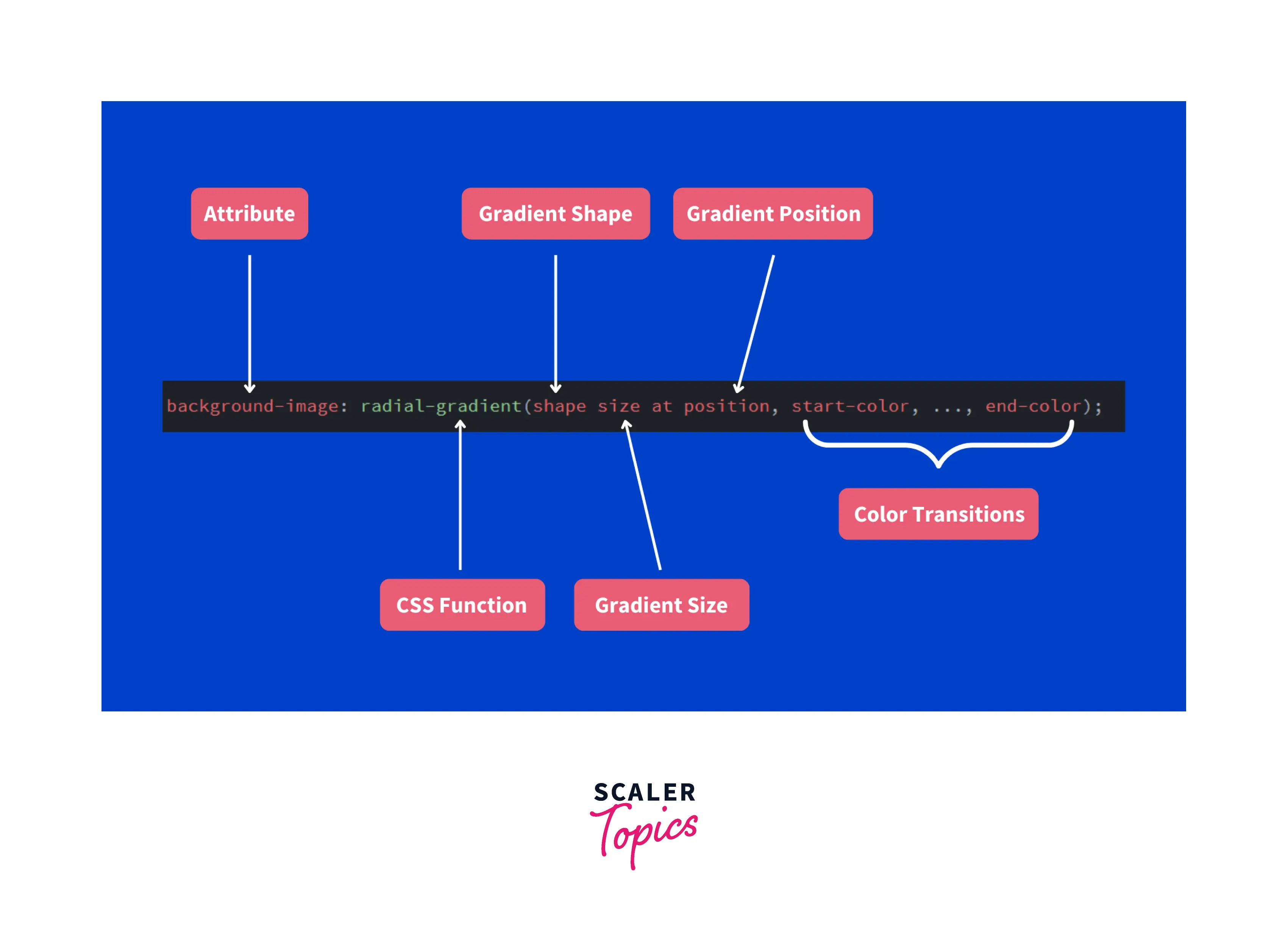Quick Example: For this example, we utilize all the parameters of a radial gradient, i.e., shape, size, position, and colors.

Result: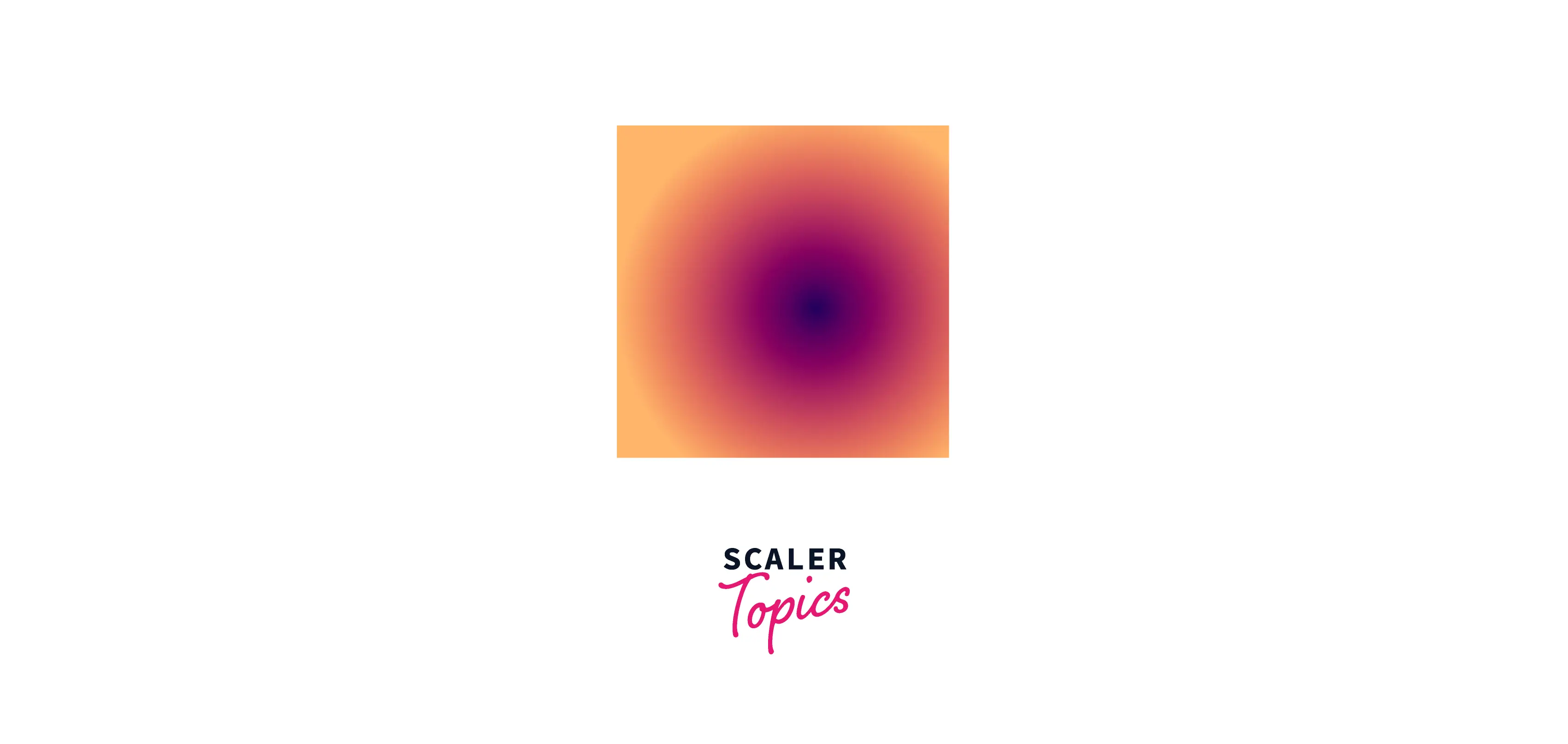### Values/Parameters

1. Shape The gradient might be elliptical or circular in shape. The default shape is an ellipse.

2. Size The gradient's size (ending shape) can be set to one of four values: farthest-corner, closest-side, closest-corner, and farthest-side. The "farthest-corner" size is the default.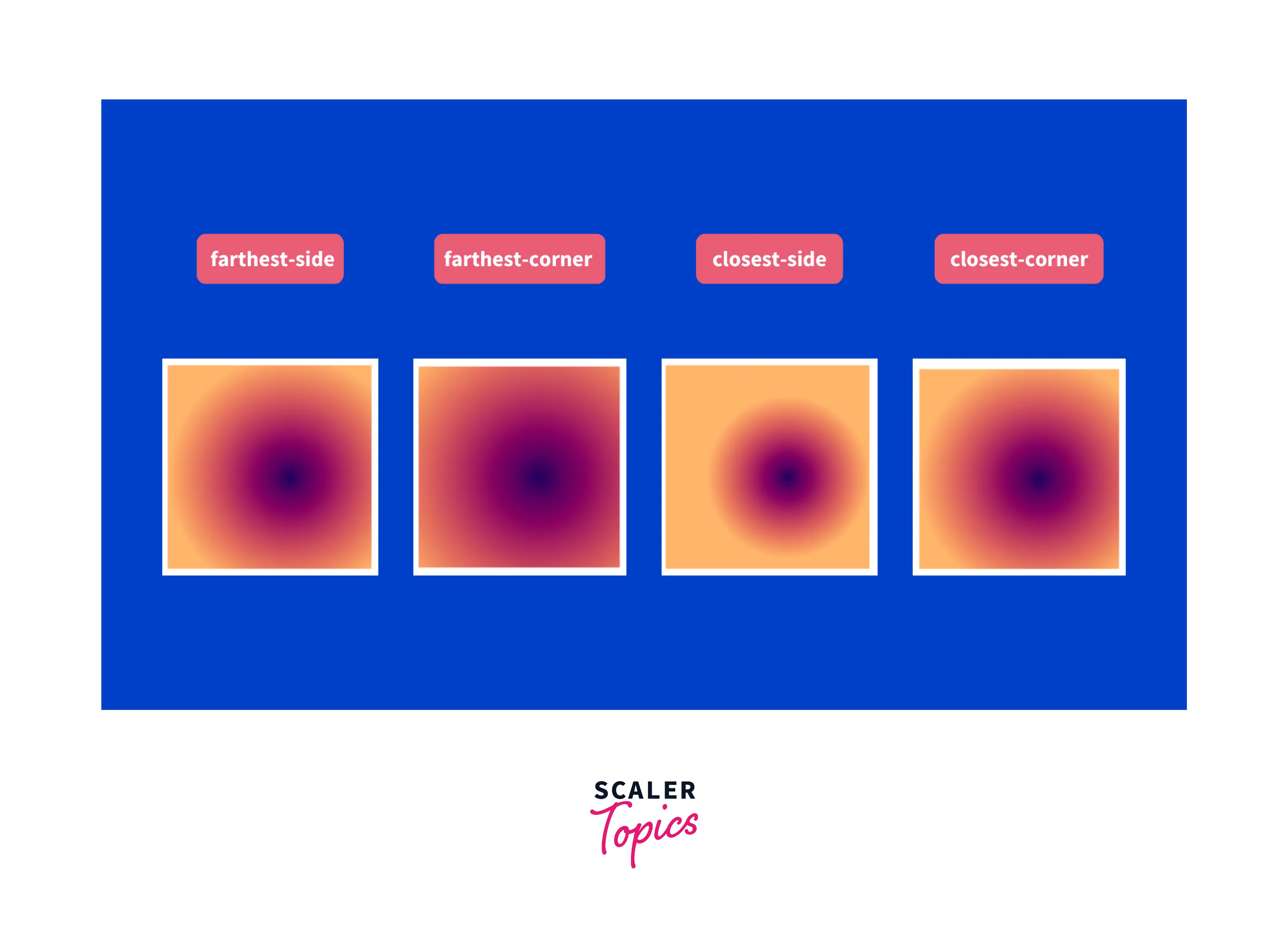3. Placement Set the gradient's location. The default setting is "center."

4. color You can specify as many colors as you want, but at least two are mandatory.

Only the beginning and ending colors are essential among these four parameters. The remaining fields are optional and have default values.

## Example

Let's create a simple gradient with default values.

HTML Create an empty div in HTML.

CSS Give that div some height and width. Include colors (at least two) in the CSS radial gradient function and leave the other parameters at their default values.

Output:The circular gradient in the output shown above is made up of the colors red (the starting color) and blue (the ending color). By default, it is centered and has the size - farthest-corner.

Let's modify the position of the gradient with the position parameter.

HTML Create an empty div in HTML.

CSS

Output: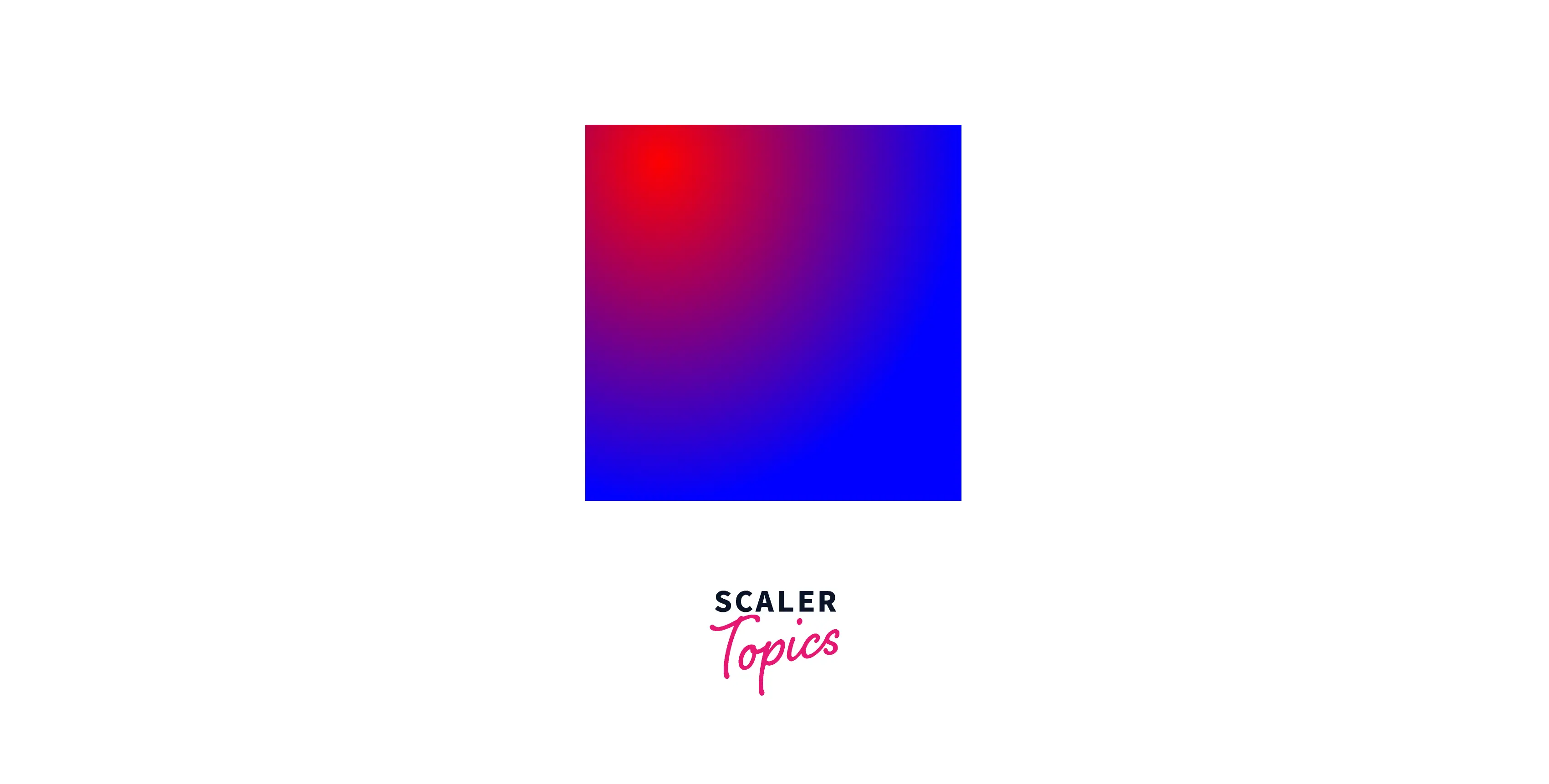As you can see in the above output, we shifted the gradient's center to 20% from the top left and 10% from the top.

### Example

HTML Create an empty div in HTML.

CSS

Output: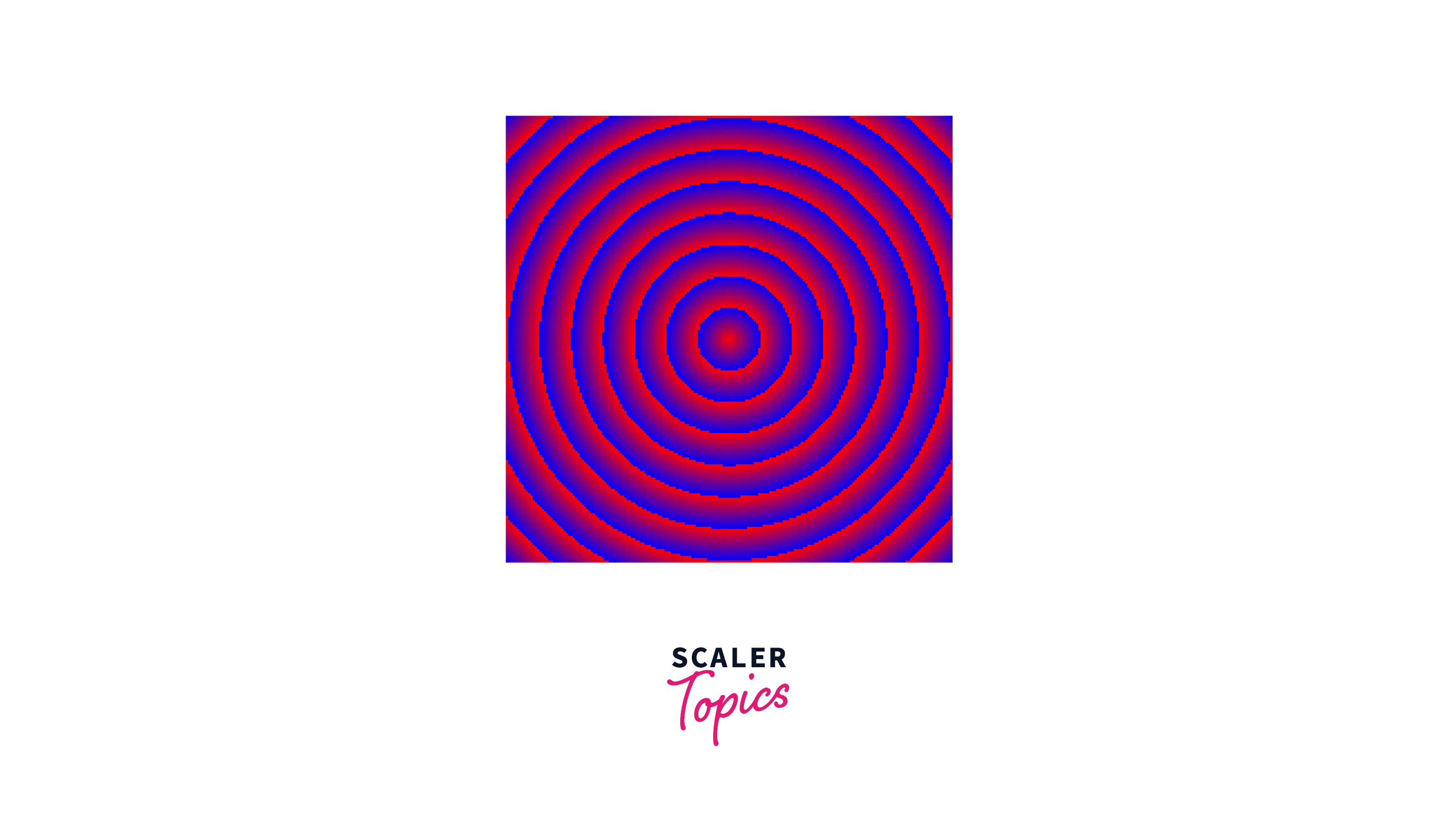Radial gradient is repeated until it reaches the container size in the output shown above.

Let's look at some patterns that you might find interesting.

### Simple pattern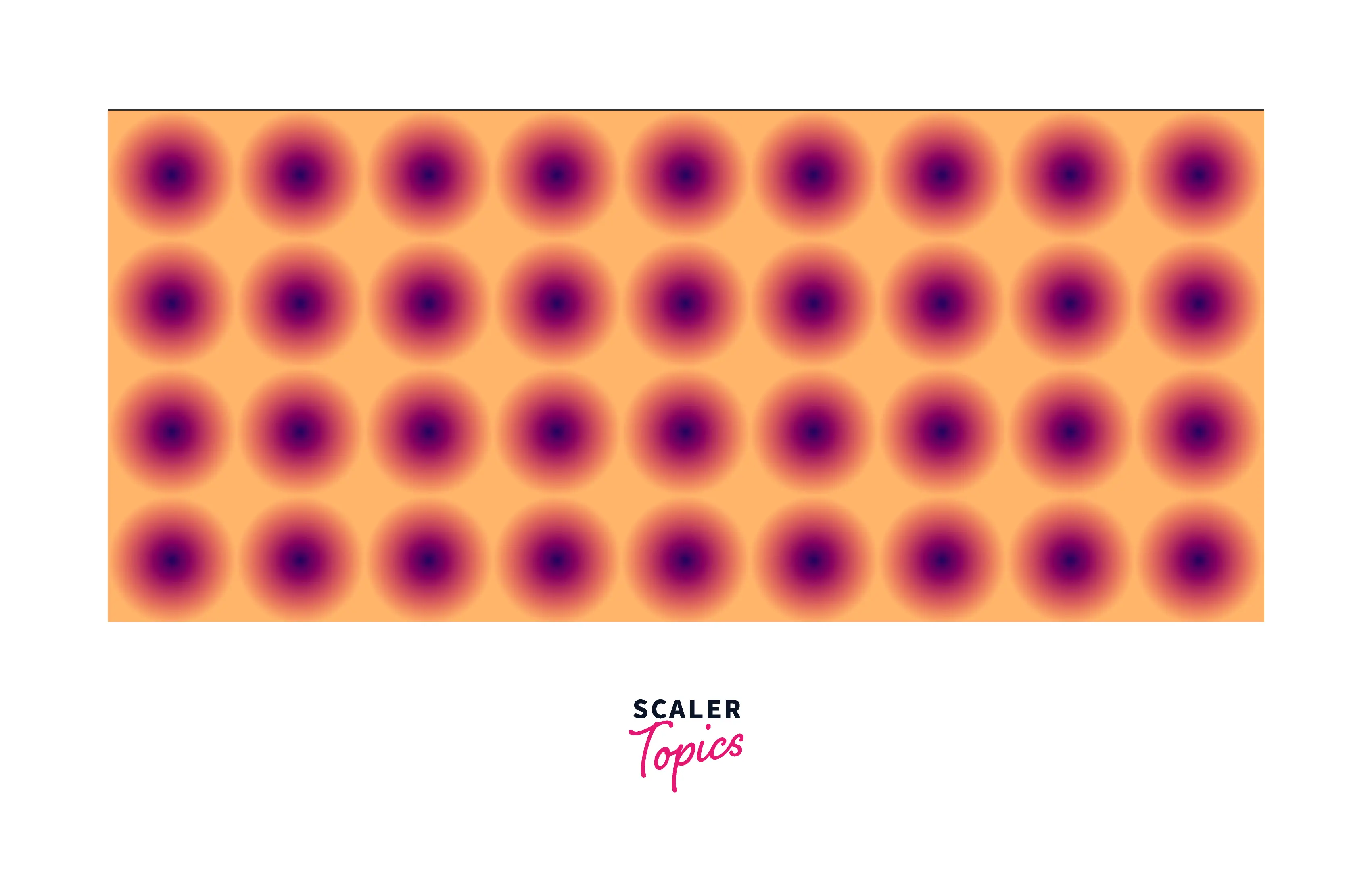Here, we give a circle a radius of 50% and set the container height to '100px.' The above pattern is produced by these two configurations.

Below is the code for the above pattern:

### Circular Zig Zag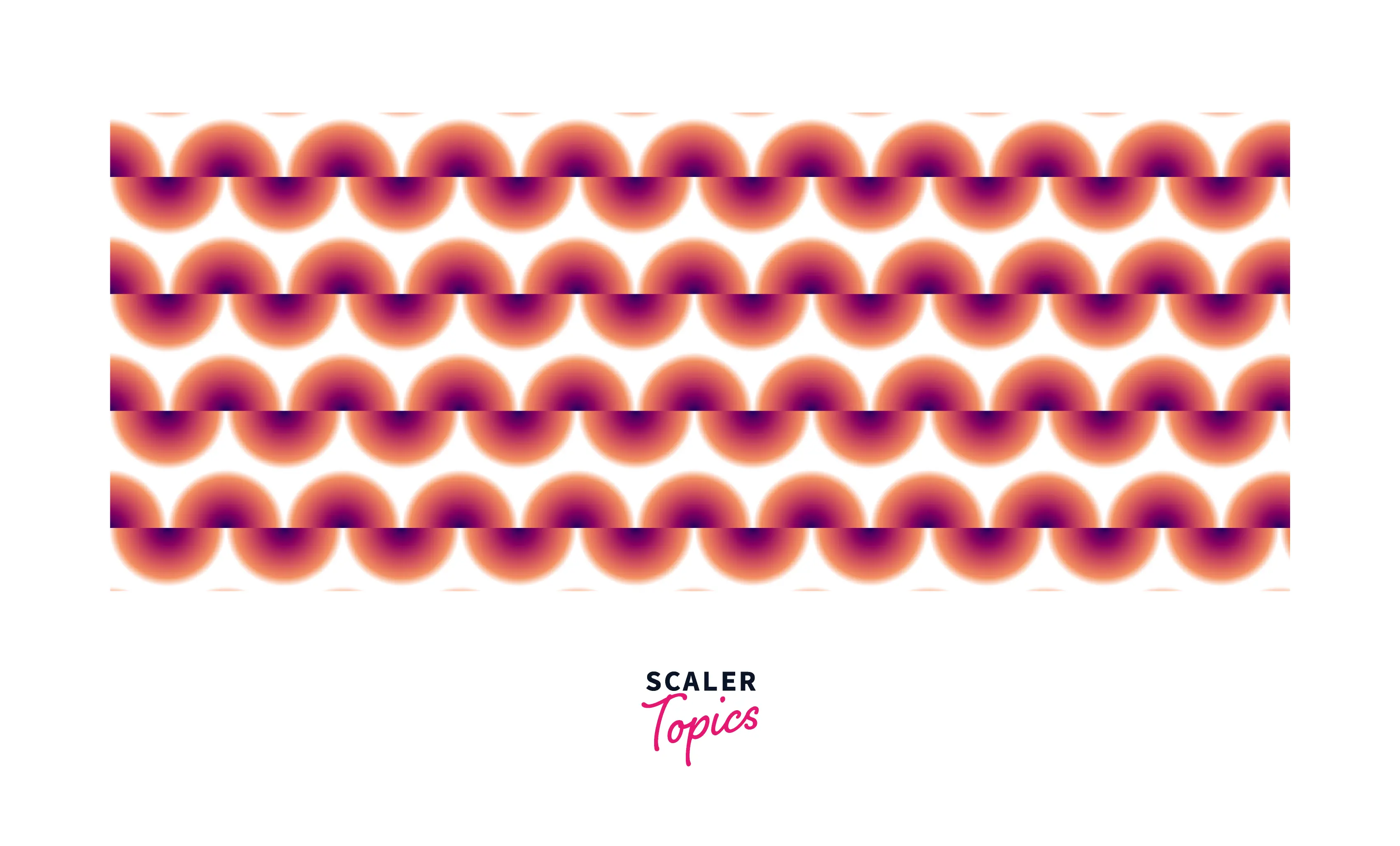Using two radial gradient css functions that overlap each other, a circular zig-zag pattern can be generated. Then we divide each gradient in half and move the second gradient by half the size of the gradient.

### Bullseye board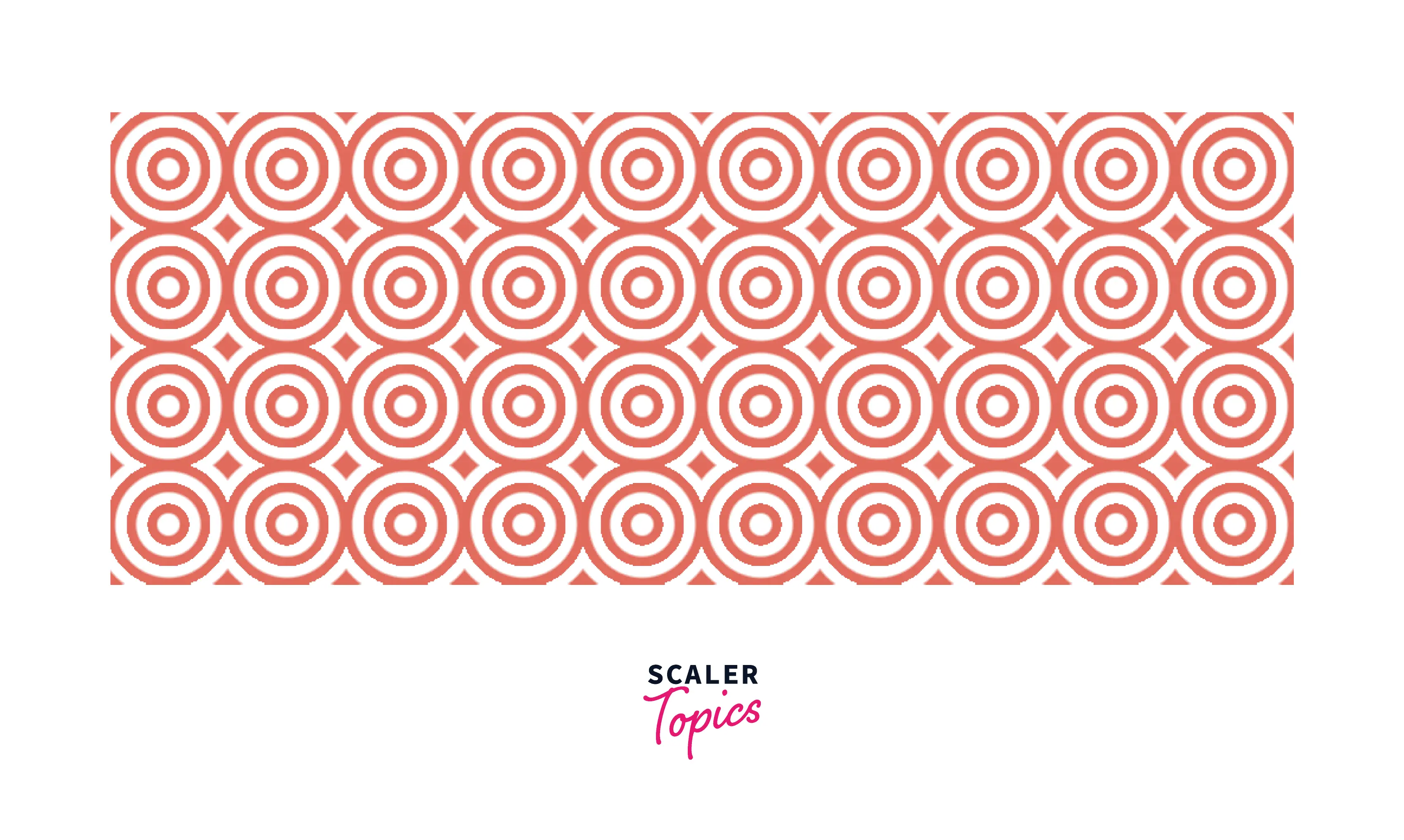The bullseye board pattern is easily created using repeating-radial-gradient() and a combination of transparent and main colors.

## More Examples

Let's see a few more examples.

### Simple Circle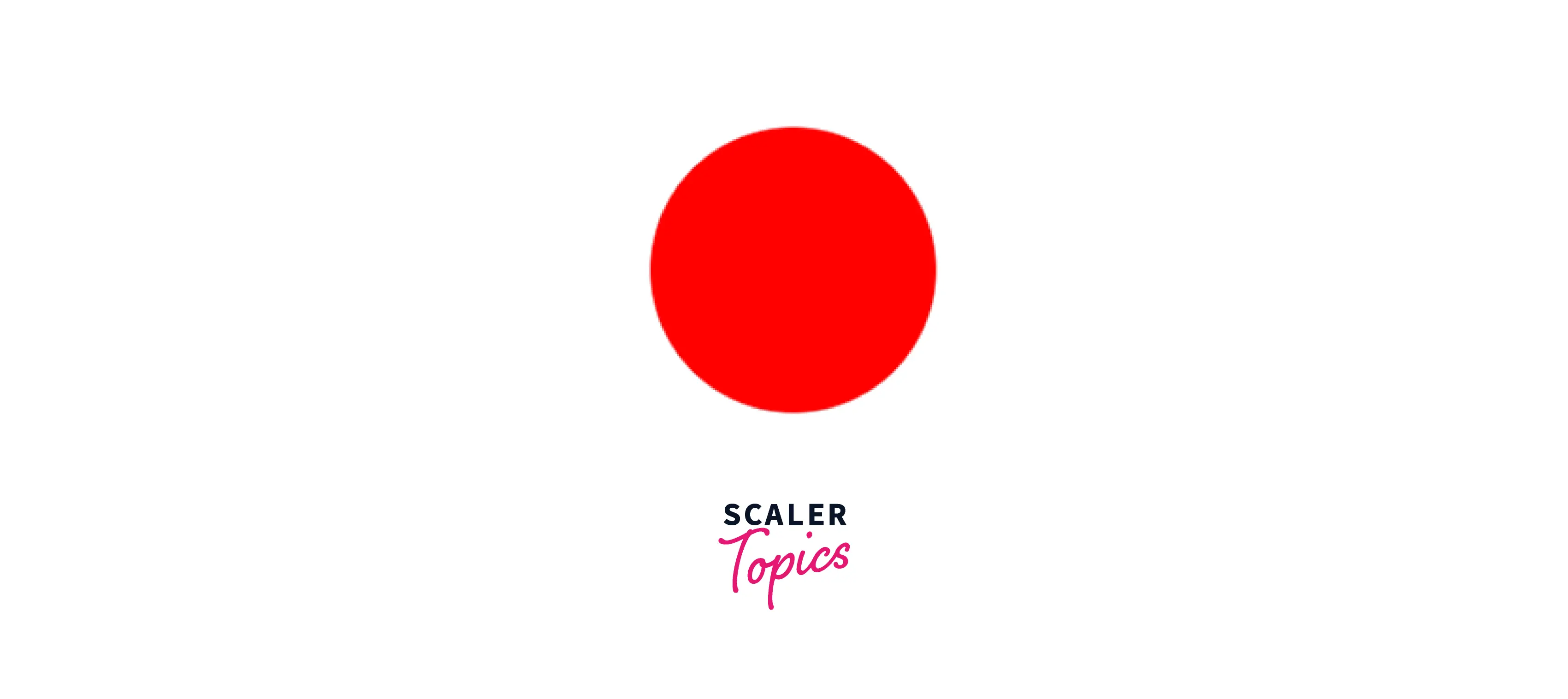By making the final color transparent, you may make a simple circle with the radial gradient css function.

### Simple Ellipse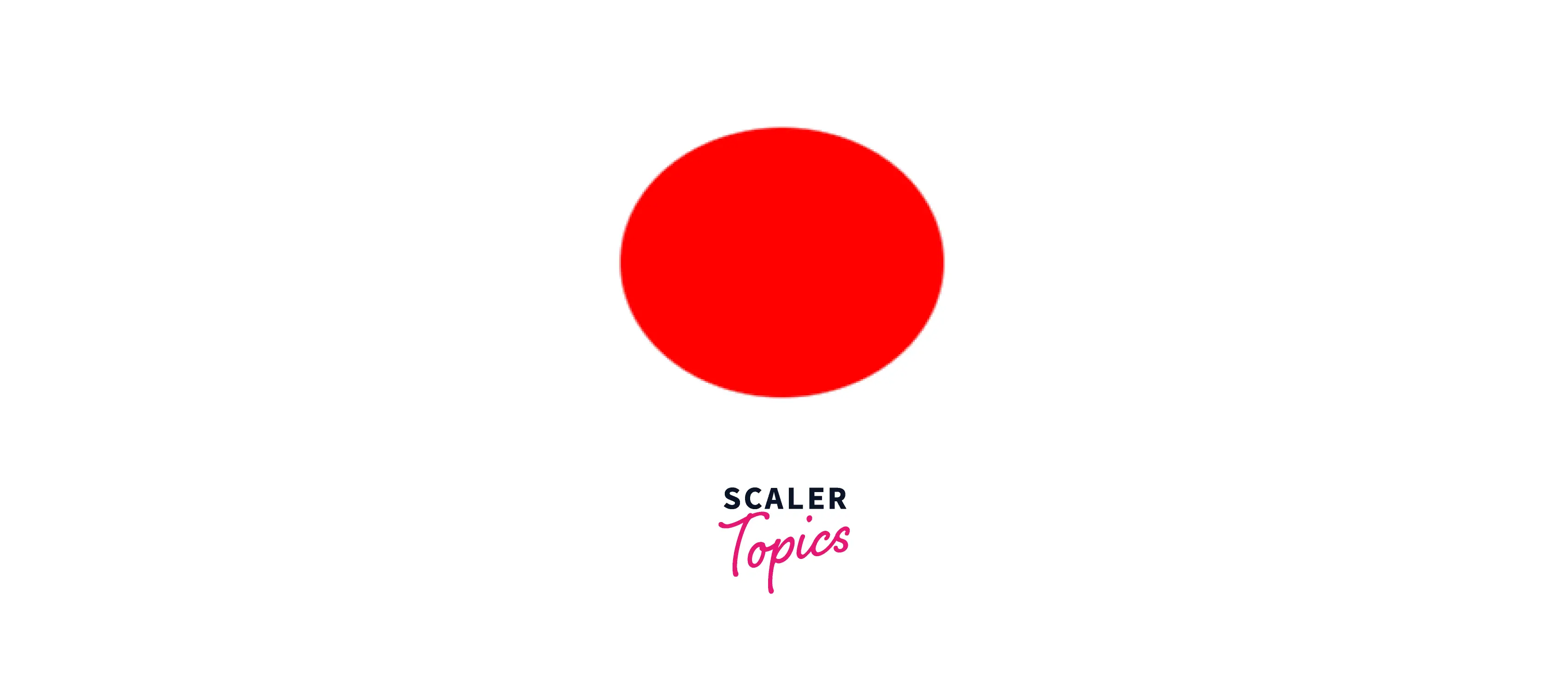You may make an ellipse by changing the shape from a circle to an ellipse.

### Evenly-spaced colors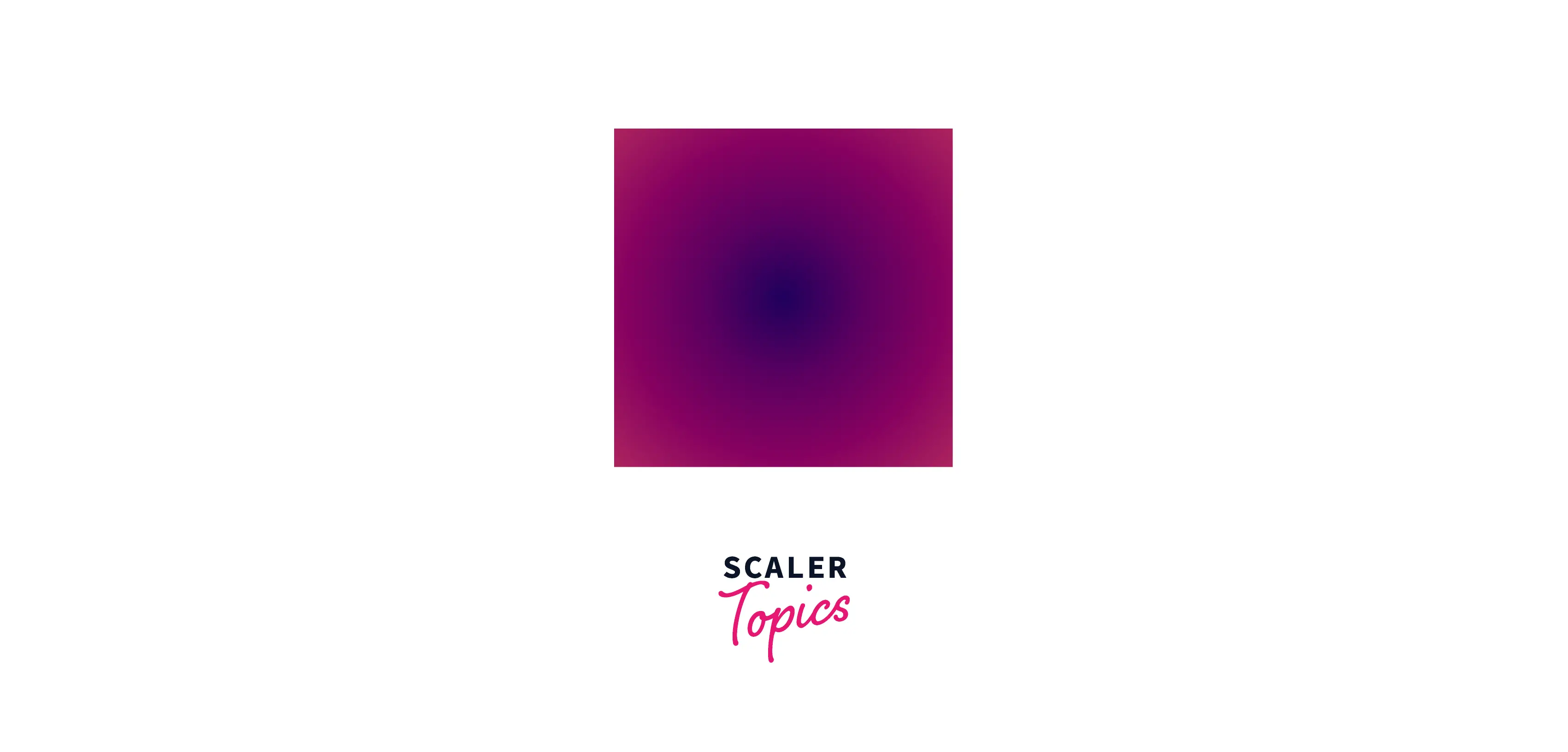Using the default values for all attributes, create an equally spaced color gradient.Conic gradients are similar to radial gradients in that color transitions occur at an angle around a center point.

## Browser Compatibility

The radial gradient css function is supported by all the popular browsers. Only the at syntax is not supported by Safari.

## Conclusion

Let us review what we have learned.

• The radial gradient function is used to create a smooth transition from one color to another.
• To use the radial gradient function in CSS, you'll need the following: a circle or container element, an image, or a combination of the two